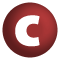# Random

#### class Random extends AnyRef

known subclasses: Random
Go to: companion

1. AnyRef
2. Any

### Value Members

1. #### def equals(arg0: Any): Boolean

This method is used to compare the receiver object (`this`) with the argument object (`arg0`) for equivalence

This method is used to compare the receiver object (`this`) with the argument object (`arg0`) for equivalence.

The default implementations of this method is an equivalence relation:

• It is reflexive: for any instance `x` of type `Any`, `x.equals(x)` should return `true`.
• It is symmetric: for any instances `x` and `y` of type `Any`, `x.equals(y)` should return `true` if and only if `y.equals(x)` returns `true`.
• It is transitive: for any instances `x`, `y`, and `z` of type `AnyRef` if `x.equals(y)` returns `true` and `y.equals(z)` returns `true`, then `x.equals(z)` should return `true`.

If you override this method, you should verify that your implementation remains an equivalence relation. Additionally, when overriding this method it is often necessary to override `hashCode` to ensure that objects that are "equal" (`o1.equals(o2)` returns `true`) hash to the same `Int` (`o1.hashCode.equals(o2.hashCode)`).

arg0

the object to compare against this object for equality.

returns

`true` if the receiver object is equivalent to the argument; `false` otherwise.

definition classes: AnyRef ⇐ Any
Go to: companion
2. #### def hashCode(): Int

Returns a hash code value for the object

Returns a hash code value for the object.

The default hashing algorithm is platform dependent.

Note that it is allowed for two objects to have identical hash codes (`o1.hashCode.equals(o2.hashCode)`) yet not be equal (`o1.equals(o2)` returns `false`). A degenerate implementation could always return `0`. However, it is required that if two objects are equal (`o1.equals(o2)` returns `true`) that they have identical hash codes (`o1.hashCode.equals(o2.hashCode)`). Therefore, when overriding this method, be sure to verify that the behavior is consistent with the `equals` method.

definition classes: AnyRef ⇐ Any
Go to: companion
3. #### def nextASCIIString(length: Int): String

Returns a pseudorandomly generated String drawing upon only ASCII characters between 33 and 126

Returns a pseudorandomly generated String drawing upon only ASCII characters between 33 and 126.

Go to: companion
4. #### def nextBoolean(): Boolean

Returns the next pseudorandom, uniformly distributed boolean value from this random number generator's sequence

Returns the next pseudorandom, uniformly distributed boolean value from this random number generator's sequence.

Go to: companion
5. #### def nextBytes(bytes: Array[Byte]): Unit

Generates random bytes and places them into a user-supplied byte array

Generates random bytes and places them into a user-supplied byte array.

Go to: companion
6. #### def nextDouble(): Double

Returns the next pseudorandom, uniformly distributed double value between 0

Returns the next pseudorandom, uniformly distributed double value between 0.0 and 1.0 from this random number generator's sequence.

Go to: companion
7. #### def nextFloat(): Float

Returns the next pseudorandom, uniformly distributed float value between 0

Returns the next pseudorandom, uniformly distributed float value between 0.0 and 1.0 from this random number generator's sequence.

Go to: companion
8. #### def nextGaussian(): Double

Returns the next pseudorandom, Gaussian ("normally") distributed double value with mean 0

Returns the next pseudorandom, Gaussian ("normally") distributed double value with mean 0.0 and standard deviation 1.0 from this random number generator's sequence.

Go to: companion
9. #### def nextInt(n: Int): Int

Returns a pseudorandom, uniformly distributed int value between 0 (inclusive) and the specified value (exclusive), drawn from this random number generator's sequence

Returns a pseudorandom, uniformly distributed int value between 0 (inclusive) and the specified value (exclusive), drawn from this random number generator's sequence.

Go to: companion
10. #### def nextInt(): Int

Returns the next pseudorandom, uniformly distributed int value from this random number generator's sequence

Returns the next pseudorandom, uniformly distributed int value from this random number generator's sequence.

Go to: companion
11. #### def nextLong(): Long

Returns the next pseudorandom, uniformly distributed long value from this random number generator's sequence

Returns the next pseudorandom, uniformly distributed long value from this random number generator's sequence.

Go to: companion
12. #### def nextString(length: Int): String

Returns a pseudorandomly generated String

Returns a pseudorandomly generated String. This routine does not take any measures to preserve the randomness of the distribution in the face of factors like unicode's variable-length encoding, so please don't use this for anything important. It's primarily intended for generating test data.

length

the desired length of the String

returns

the String

Go to: companion

15. #### def toString(): String

Returns a string representation of the object

Returns a string representation of the object.

The default representation is platform dependent.

definition classes: AnyRef ⇐ Any
Go to: companion

### Instance constructors

1. #### new Random()

Creates a new random number generator

Creates a new random number generator.

Go to: companion
2. #### new Random(seed: Int)

Creates a new random number generator using a single integer seed

Creates a new random number generator using a single integer seed.

Go to: companion
3. #### new Random(seed: Long)

Creates a new random number generator using a single long seed

Creates a new random number generator using a single long seed.

Go to: companion